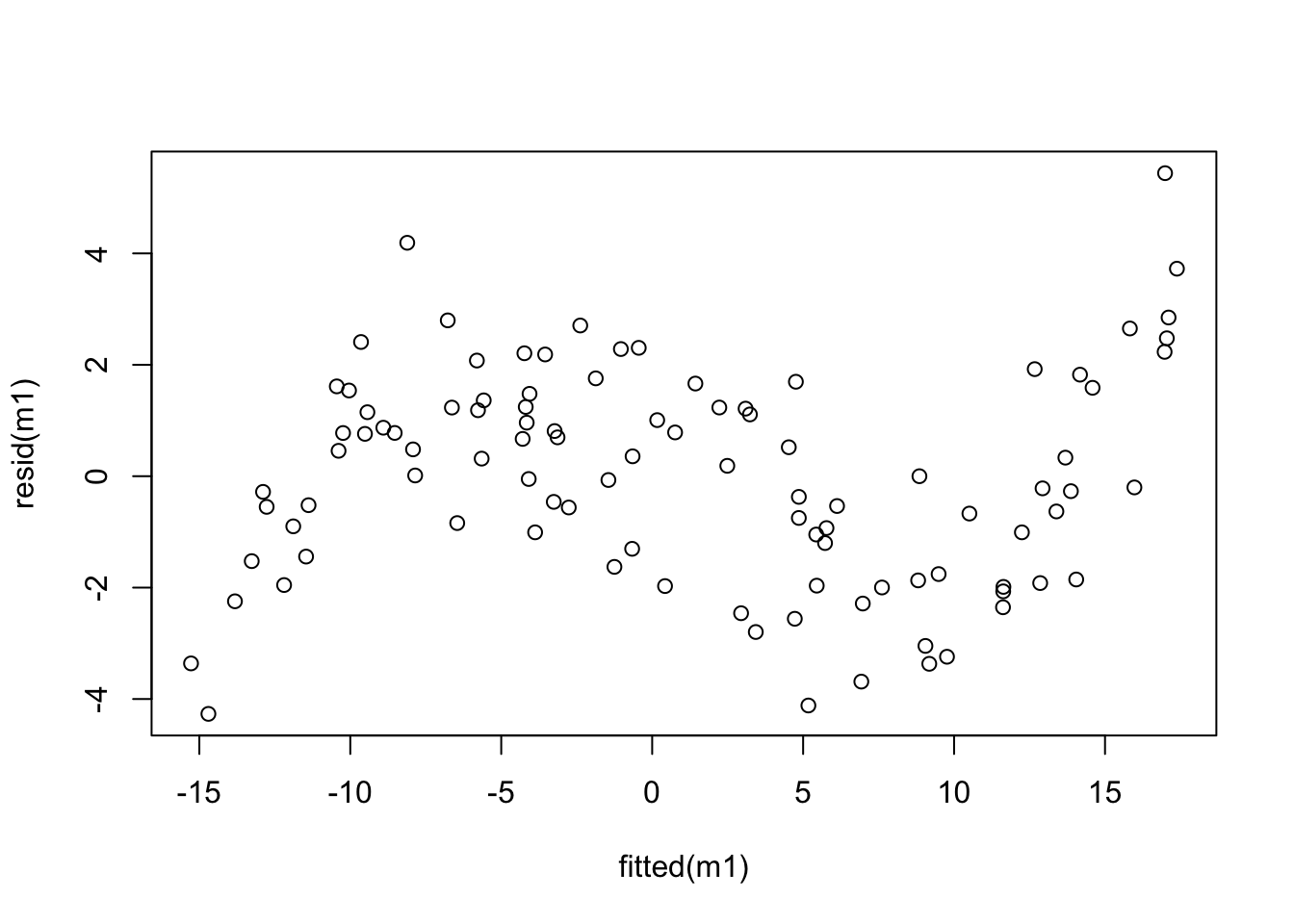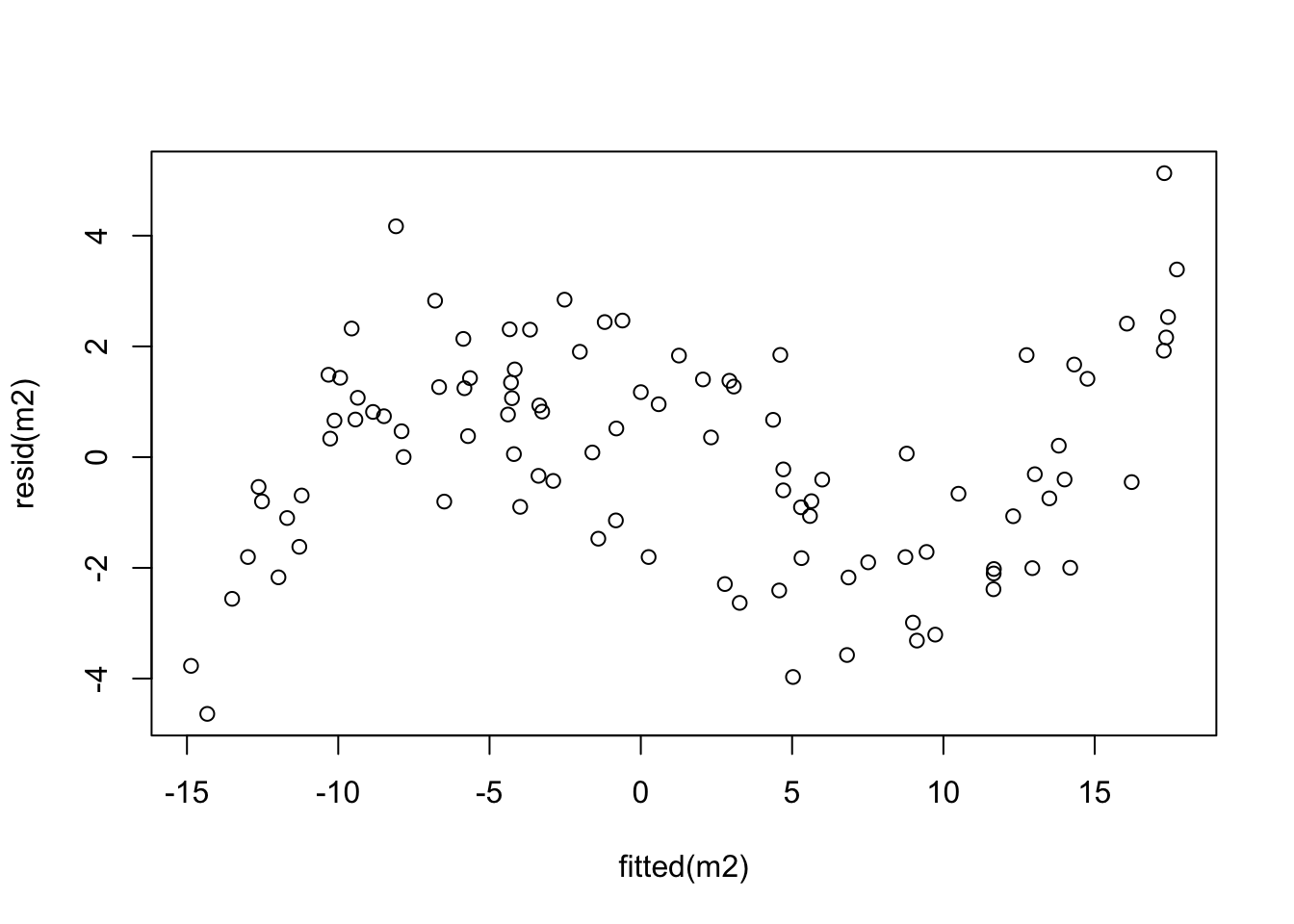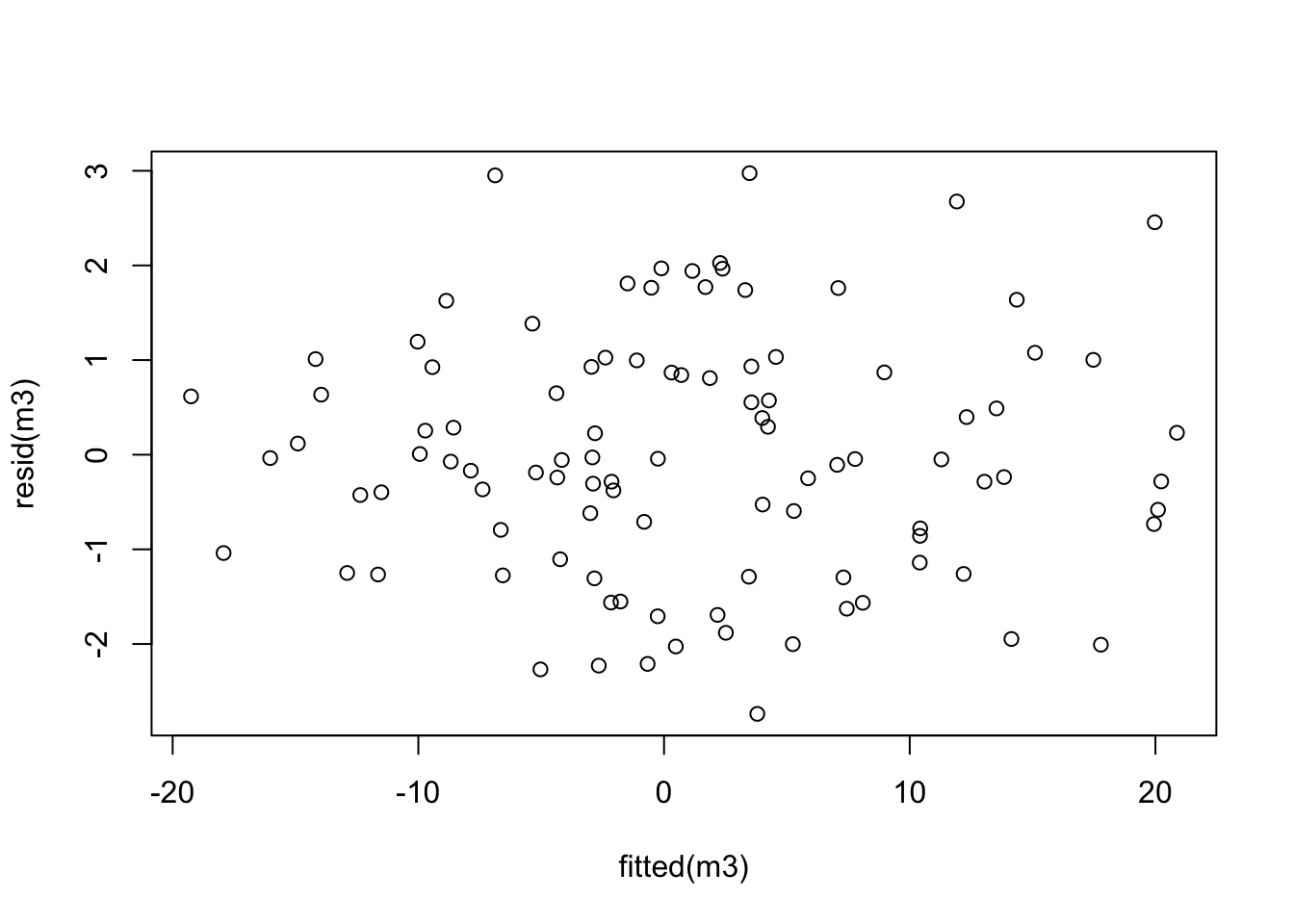# Problem Sheet 2

You should attempt all these questions and write up your solutions in advance of the workshop in week 4 where the answers will be discussed.

5. Assume that $$X \sim \mathcal{N}(0, 1)$$, $$Y\sim \chi^2(2)$$ and $$Z \sim \chi^2(3)$$ are independent. What are the distributions of the following random variables?

• $$X^2$$,

• $$X^2 + Y + Z$$,

• $$\displaystyle\frac{\sqrt{2}X}{\sqrt{Y}}$$,

• $$\displaystyle\frac{2X^2}{Y}$$, and

• $$1.5\, Y/Z$$.

The distributions involved in this question are the normal distribution, the $$\chi^2$$-dis-tri-bu-tion, the $$t$$-distribution and the $$F$$-distribution. The general rules are: (1) the sum of squares of $$d$$ independent, standard normally distributed random variables follows a $$\chi^2(d)$$-distribution. (2) If $$Z\sim\mathcal{N}(0,1)$$ and $$V \sim \chi^2(d)$$ are independent, then $$Z / \sqrt{V / d} \sim t(d)$$, and finally (3) If $$V_1\sim \chi^2(d_1)$$ and $$V_2\sim \chi^2(d_2)$$ are independent, then $$(V_1/d_1)/(V_2/d_2) \sim F(d_1, d_2)$$. Using these rules:

• $$X^2 \sim \chi^2(1)$$,

• $$X^2 + Y + Z \sim \chi^2(1+2+3) = \chi^2(6)$$,

• $$\displaystyle\frac{\sqrt{2}X}{\sqrt{Y}} = \frac{X}{\sqrt{Y/2}} \sim t(2)$$,

• $$\displaystyle\frac{2X^2}{Y} = \frac{X^2/1}{Y/2} \sim F(1,2)$$,

• $$\displaystyle 1.5\, Y/Z = \frac{Y/2}{Z/3} \sim F(2, 3)$$.

6. Let $$\mathbf{1} = (1, 1, \ldots, 1) \in\mathbb{R}^n$$ and let $$X \sim \mathcal{N}(\mu, \sigma^2 I)$$ be a normally-distributed random vector, where $$I$$ is the $$n\times n$$ identity matrix. Define $$A = \frac1n \mathbf{1} \mathbf{1}^\top \in \mathbb{R}^{n\times n}$$.

• Show that $$(AX)_i = \bar X$$ for all $$i \in \{1, \ldots, n\}$$, where $$\bar X = \frac1n \sum_{j=1}^n X_j$$.

• Show that $$A$$ is symmetric.

• Show that $$A$$ is idempotent.

• Use results from lectures to conclude that the random variables $$AX$$ and $$X^\top (I-A)X$$ are independent.

• Using the previous parts of the question, show that $$\bar X$$ and $$\frac{1}{n-1} \sum_{i=1}^n (X_i - \bar X)^2$$ are independent.

The matrix $$A = (a_{ij})$$ has entries $$a_{ij} = 1/n$$ for all $$i, j \in \{1, \ldots, n\}$$. Using this observation, the statements of the first few questions follow easily:

• We have $\begin{equation*} (Ax)_i = \sum_{j=1}^n a_{ij} x_j = \sum_{j=1}^n \frac1n x_j = \frac1n \sum_{j=1}^n x_j = \bar x \end{equation*}$ for all $$i \in \{1, \ldots, n\}$$.

• We have $\begin{equation*} a_{ij} = \frac1n = a_{ji} \end{equation*}$ for all $$i, j \in \{1, \ldots, n\}$$ and thus $$A$$ is symmetric.

• We have $$\mathbf{1}^\top \mathbf{1} = \sum_{i=1}^n 1 \cdot 1 = n$$ and thus $\begin{equation*} A^2 = \bigl( \frac1n \mathbf{1} \mathbf{1}^\top \bigr) \bigl( \frac1n \mathbf{1} \mathbf{1}^\top \bigr) = \frac{1}{n^2} \mathbf{1} \bigl(\mathbf{1}^\top\mathbf{1}\bigr) \mathbf{1}^\top = \frac1n \mathbf{1} \mathbf{1}^\top = A. \end{equation*}$ (Alternatively, we could use the fact that we know the entries of $$A$$ and compute $$A^2$$ explicitly.)

• For the remaining statements we will use the fact that $$X$$ is normally distributed. We will repeatedly use the result that if two random variables $$X$$ and $$Y$$ are independent, that then any function of $$X$$ is independent of any function of $$Y$$.

In lectures we learned that, if $$A$$ is symmetric and idempotent and if $$\varepsilon\sim \mathcal{N}(0, I)$$, then $$A\varepsilon$$ and $$(I-A)\varepsilon$$ are independent. Applying this result with $$\varepsilon= (X - \mu \mathbf{1}) / \sigma$$ we find that $$AX = \sigma A\varepsilon+ \mu A \mathbf{1}$$ and $$(I-A)X = \sigma (I-A)\varepsilon+ \mu (I-A)\mathbf{1}$$ are independent, since they are functions of $$A\varepsilon$$ and $$(I-A)\varepsilon$$.

Since $$(I - A)^\top (I - A) = I^2 - I A - A I + A^2 = I - A - A + A = I - A$$ we have $\begin{equation*} X^\top (I-A)X = X^\top (I - A)^\top (I-A)X = \Bigl( (I-A)X \Bigr)^\top \Bigl( (I-A)X \Bigr). \end{equation*}$ Thus, $$X^\top (I-A)X$$ is a function of $$(I-A)X$$ and as such is also independent of $$AX$$.

• We have seen $$(AX)_i = \bar X$$ and thus $$\bigl((I - A)X\bigr)_i = X_i - \bar X$$. This gives \begin{align*} X^\top (I-A)X &= \Bigl( (I-A)X \Bigr)^\top \Bigl( (I-A)X \Bigr) \\ &= \sum_{i=1}^n \Bigl( (I-A)X \Bigr)_i^2 \\ &= \sum_{i=1}^n (X_i - \bar X)^2 \end{align*} Thus we can write the sample mean as $$\bar X = (AX)_i$$, and the sample variance as $$\mathrm{s}_X^2 = \frac{1}{n-1} \sum_{i=1}^n (X_i - \bar X)^2 = X^\top (I-A)X / (n-1)$$, and if $$X$$ is normally distributed the two values $$\bar X$$ and $$\mathrm{s}_X^2$$ are independent.

7. Consider the following R commands:

m <- lm(stack.loss ~ ., data=stackloss)
summary(m)

Call:
lm(formula = stack.loss ~ ., data = stackloss)

Residuals:
Min      1Q  Median      3Q     Max
-7.2377 -1.7117 -0.4551  2.3614  5.6978

Coefficients:
Estimate Std. Error t value Pr(>|t|)
(Intercept) -39.9197    11.8960  -3.356  0.00375 **
Air.Flow      0.7156     0.1349   5.307  5.8e-05 ***
Water.Temp    1.2953     0.3680   3.520  0.00263 **
Acid.Conc.   -0.1521     0.1563  -0.973  0.34405
---
Signif. codes:  0 '***' 0.001 '**' 0.01 '*' 0.05 '.' 0.1 ' ' 1

Residual standard error: 3.243 on 17 degrees of freedom
Multiple R-squared:  0.9136,    Adjusted R-squared:  0.8983
F-statistic:  59.9 on 3 and 17 DF,  p-value: 3.016e-09

Either using this output, or using R to further inspect the built-in stackloss dataset, find a $$99\%$$-confidence interval for the parameter $$\beta_\mathtt{Acid.Conc.}$$.

From lectures, we know that a confidence interval for a single coefficient $$\beta_j$$ is given by $\begin{equation*} [U, V] = \Bigl[ \hat\beta_j - t_{n-p-1}(\alpha/2) \sqrt{\widehat{\sigma^2} C_{jj}}, \hat\beta_j + t_{n-p-1}(\alpha/2) \sqrt{\widehat{\sigma^2} C_{jj}} \Bigr], \end{equation*}$ where $$t_{n-p-1}$$ is the $$(1-\alpha/2)$$-quantile of the $$t$$-distribution, $$C_{jj} = (X^\top X)^{-1}_{jj}$$, and $$X$$ is the design matrix.

We can read off the required values for computing the confidence interval from the output of summary(m): the centre of the confidence interval can be found in the column Estimate, the standard error $$\sqrt{\widehat{\sigma^2} C_{jj}}$$ is given in column Std. Error, and the value $$n-p-1$$ for the $$t$$-quantile can be found as the degrees of freedom for the residual standard error, near the bottom of the output. Using these values, we can find a $$95\%$$-confidence interval for Acid.Conc. as follows:

c(-0.1521 - qt(0.995, 17) * 0.1563, -0.1521 + qt(0.995, 17) * 0.1563)
 -0.6050934  0.3008934

Thus, the confidence interval is $$\bigl[ -0.605, 0.301 \bigr]$$.

8. The file 20211020.csv from

contains pairs of $$(x, y)$$ values. Fit the following models to the data:

• $$y = \beta_0 + \beta_1 x + \varepsilon$$

• $$y = \beta_0 + \beta_1 x + \beta_2 x^2 + \varepsilon$$

• $$y = \beta_0 + \beta_1 x + \beta_2 x^2 + \beta_3 x^3 + \varepsilon$$

For each model, create a “residual plot”, i.e. a scatter plot which has the fitted values on the horizontal axis and the residuals on the vertical axis. Comment on the resulting plots.

# data from https://www1.maths.leeds.ac.uk/~voss/data/20211020.csv

m1 <- lm(y ~ x, data = d)
plot(fitted(m1), resid(m1))m2 <- lm(y ~ x + I(x^2), data = d)
plot(fitted(m2), resid(m2))m3 <- lm(y ~ x + I(x^2) + I(x^3), data = d)
plot(fitted(m3), resid(m3))